### Home > CALC > Chapter 7 > Lesson 7.3.1 > Problem7-113

7-113.
1. Simplify these exponential expressions. Try each without a calculator first. Homework Help ✎

1. eln(11)

2. ln(e4)

3. ln(ee )

4. 10log(x)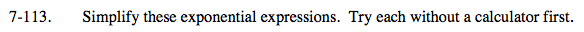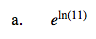Translation: Raise e to the power of something whose exponent makes e equal 11.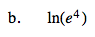Power Law of Logarithms.

4lne = 4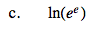Refer to the hint in part (b).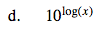If the base of a logarithm is not given, then assume that the base is 10. Refer to the hint in part (a) for more guidance.